# [Ruby] See the essence of ArgumentError

ArgumentError Argument means "argument". ArgumentError will be the message "The number of arguments does not match". Various cases are possible, so Let's consider the essential basic part as an example.

Introduced on the wiki as "a shell for running Ruby interactively (REPL)" I will use irb.

Execute code using the following actual and formal arguments with irb

``````def test(number)
puts number * number
end

test(5)
``````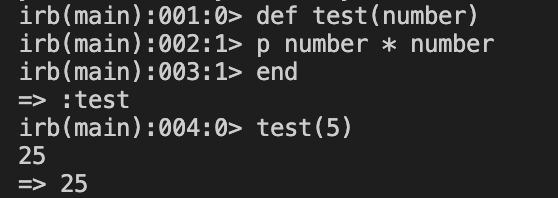If you set (5) to the argument of test and pass a value to the formal argument (number), The result of 25 was output.

Now let's run test with no actual arguments.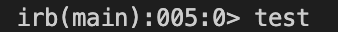Then an error occurred.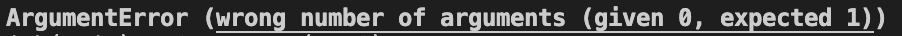Here is the translation of the error message: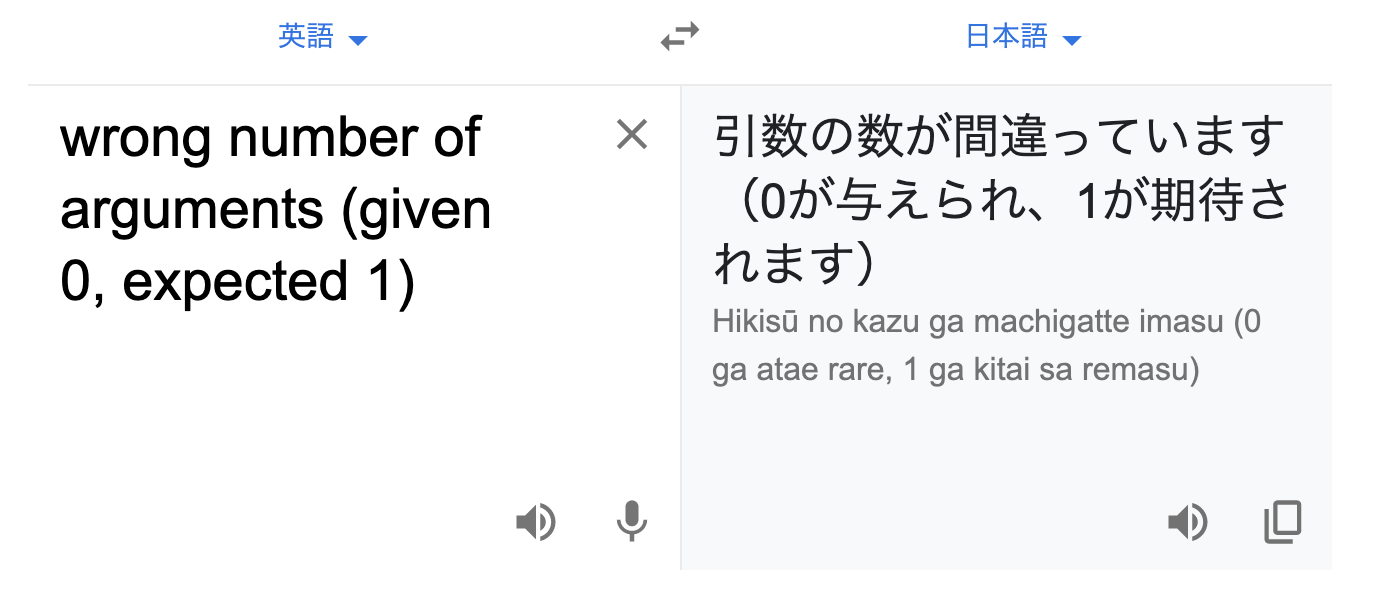The computer says "I expected it to have one argument, but it was 0." I'm missing one argument.

Now, let's execute it with two actual arguments.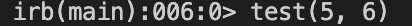This time you say "I expected it to have one argument, but it was two." I'm angry that there is one more.

Even if you try to send two actual arguments (5, 6), it will not work if the number of formal arguments (number) on the receiving side is one.

# Summary

ArgumentError is an error saying "The number of arguments does not match". Let's check the actual and formal arguments where the method is defined!